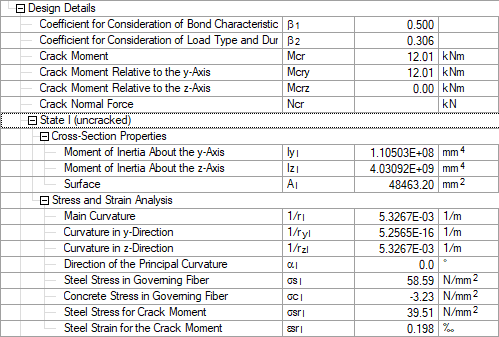# RF-CONCRETE Members – Online Manual Version 5

Online manuals, introductory examples, tutorials, and other documentation.

# 9.2.6.2 State I (uncracked)

#### State I (uncracked)

When determining the cross-section properties, we take the available steel area into account. The missing area of concrete in the zone of rebars is neglected. Recalculating the centroid of the ideal cross-section is not necessary because the reinforcement is symmetric with the same edge distances on top and bottom side.

The following distances for the Steiner component (parallel axis theorem) are the direct result:

• ac = 0 cm
• as1 = 8 − 2.5 = 5.5 cm
• as2 = 5.5 cm
Moment of inertia

Ideal cross-section area

Crack moment Mcr

We assume that the cross-section cracks when the tensile strength fctm in the most external fiber is reached.

Steel stress σsrI and steel strain εsrI for crack moment

Notional steel and concrete stress for effective moment M = 17.64 kNm

Curvature for uncracked section (state I) (1/r)z,I = (1/r)I

Results of RF-CONCRETE MembersFigure 9.23 Detailed results for state I